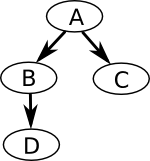Practice Exercise 6.E
Approximate Inference

## 2: Learning Goals

• Determine the variable ordering needed to use forward sampling on a Bayesian network.
• Apply concepts from the rejection sampling algorithm to a simple problem.
• Apply concepts from the importance sampling algorithm to a simple problem.
• Apply concepts from particle filtering to a simple problem.

## 3: Directed Questions

1. When would approximate inference be preferable to exact inference techniques such as variable elimination? [solution]

2. You randomly throw 200 darts at a dartboard (the probability of hitting any spot on the dartboard is uniform). The dartboard has two types of regions: red and black. 57 of the darts you threw hit a red region. Approximately what percentage of the dartboard is black? [solution]

3. Suppose you are sampling from a single variable and trying to estimate the probability of a particular value of that variable. Using Hoeffding's inequality, how many samples would you need in order to get an error of less than 0.05 in 90% of cases? [solution]

## 4: Exercise: Approximate Inference

Consider the following belief network:Define the conditional probability table of node B by P(b|a) = 0.0015, P(b|¬a) = 0.56. In addition, assume P(b,c)=0.2.
Note: we sometimes use a shorthand notation for boolean variables. For example, P(b|¬a) is equivalent to P(B = true | A = false).
1. How many possible variable orderings would there be if we were to perform forward sampling in this network? [solution]

2. We can estimate P(a|b,c) using rejection sampling. If 1,000 samples are taken, how many samples do you expect will be rejected? [solution]

3. We can estimate P(d|a) using rejection sampling. If 2,000 samples are not rejected, approximately how many samples will have both a and b true? [solution]

4. Suppose now we use importance sampling, with the proposal distribution Q(b|a) = 0.4. Now, among the 2,000 samples with A=true, how many will be expected to have A=B=true? What are the weights of these samples? What are the weights of samples with A=true, B=false? [solution]

5. Suppose we use particle filtering and have a particle with the partial assignment A=false, C=true, and weight 0.25. After absorbing the evidence B=false, what is the particle's new weight? [solution]

6. After sampling A and C and absorbing ¬b in a population of 1,000 particles, suppose the total weight of all particles is 27.5. If we now resample the population, about how many copies of the particle described in the previous question will we end up with? [solution]

## 5: Learning Goals Revisited

• Determine the variable ordering needed to use forward sampling on a Bayesian network.
• Apply concepts from the rejection sampling algorithm to a simple problem.
• Apply concepts from the importance sampling algorithm to a simple problem.
• Apply concepts from particle filtering to a simple problem.